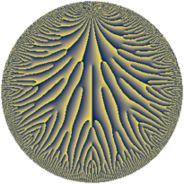# Properties

 Label 16.48.a.dLevel $16$ Weight $48$ Character orbit 16.a Self dual yes Analytic conductor $223.852$ Analytic rank $1$ Dimension $4$ CM no Inner twists $1$

# Learn more

## Newspace parameters

 Level: $$N$$ $$=$$ $$16 = 2^{4}$$ Weight: $$k$$ $$=$$ $$48$$ Character orbit: $$[\chi]$$ $$=$$ 16.a (trivial)

## Newform invariants

 Self dual: yes Analytic conductor: $$223.852260248$$ Analytic rank: $$1$$ Dimension: $$4$$ Coefficient field: $$\mathbb{Q}[x]/(x^{4} - \cdots)$$ Defining polynomial: $$x^{4} - x^{3} - 832803191366 x^{2} + 3710135215485780 x + 13175318942671469337000$$ Coefficient ring: $$\Z[a_1, \ldots, a_{5}]$$ Coefficient ring index: $$2^{46}\cdot 3^{7}\cdot 5^{3}\cdot 7^{2}$$ Twist minimal: no (minimal twist has level 1) Fricke sign: $$-1$$ Sato-Tate group: $\mathrm{SU}(2)$

## $q$-expansion

Coefficients of the $$q$$-expansion are expressed in terms of a basis $$1,\beta_1,\beta_2,\beta_3$$ for the coefficient ring described below. We also show the integral $$q$$-expansion of the trace form.

 $$f(q)$$ $$=$$ $$q + ( -9615373740 - \beta_{1} ) q^{3} + ( -7778670060568050 + 143248 \beta_{1} - 47 \beta_{2} - 3 \beta_{3} ) q^{5} + ( 9792304681472105800 + 288647031 \beta_{1} - 2171627 \beta_{2} + 4180 \beta_{3} ) q^{7} + ( -4267993104339535550043 - 69250029588 \beta_{1} + 301730130 \beta_{2} - 1508274 \beta_{3} ) q^{9} +O(q^{10})$$ $$q +(-9615373740 - \beta_{1}) q^{3} +(-7778670060568050 + 143248 \beta_{1} - 47 \beta_{2} - 3 \beta_{3}) q^{5} +(9792304681472105800 + 288647031 \beta_{1} - 2171627 \beta_{2} + 4180 \beta_{3}) q^{7} +(-$$$$42\!\cdots\!43$$$$- 69250029588 \beta_{1} + 301730130 \beta_{2} - 1508274 \beta_{3}) q^{9} +($$$$47\!\cdots\!28$$$$- 3164814174665 \beta_{1} + 43653145690 \beta_{2} + 217326120 \beta_{3}) q^{11} +($$$$31\!\cdots\!30$$$$+ 428253881669304 \beta_{1} + 636735319961 \beta_{2} - 14610507235 \beta_{3}) q^{13} +(-$$$$31\!\cdots\!00$$$$+ 9683060038655529 \beta_{1} + 12264444450819 \beta_{2} + 468532892556 \beta_{3}) q^{15} +($$$$52\!\cdots\!70$$$$- 231250822893144132 \beta_{1} - 567367762804830 \beta_{2} - 5862494129970 \beta_{3}) q^{17} +($$$$26\!\cdots\!60$$$$- 6399332107287301719 \beta_{1} - 9378200637116970 \beta_{2} - 21154099092072 \beta_{3}) q^{19} +(-$$$$65\!\cdots\!28$$$$+ 56460333251491679048 \beta_{1} - 98870608738814220 \beta_{2} + 903384551930364 \beta_{3}) q^{21} +(-$$$$34\!\cdots\!20$$$$+ 38416206999361792313 \beta_{1} - 579467257376567677 \beta_{2} + 151096756188300 \beta_{3}) q^{23} +($$$$25\!\cdots\!75$$$$+$$$$25\!\cdots\!00$$$$\beta_{1} - 11090484330999303100 \beta_{2} - 67016296066966900 \beta_{3}) q^{25} +($$$$18\!\cdots\!80$$$$+$$$$16\!\cdots\!56$$$$\beta_{1} + 39260056568752771362 \beta_{2} - 96744589719397560 \beta_{3}) q^{27} +(-$$$$56\!\cdots\!90$$$$-$$$$46\!\cdots\!04$$$$\beta_{1} -$$$$31\!\cdots\!55$$$$\beta_{2} + 2665048030584989913 \beta_{3}) q^{29} +(-$$$$18\!\cdots\!12$$$$-$$$$11\!\cdots\!20$$$$\beta_{1} +$$$$37\!\cdots\!20$$$$\beta_{2} + 9951638514076833760 \beta_{3}) q^{31} +($$$$65\!\cdots\!80$$$$-$$$$59\!\cdots\!48$$$$\beta_{1} -$$$$42\!\cdots\!10$$$$\beta_{2} - 37812601030217045670 \beta_{3}) q^{33} +($$$$32\!\cdots\!00$$$$-$$$$47\!\cdots\!88$$$$\beta_{1} +$$$$55\!\cdots\!32$$$$\beta_{2} -$$$$28\!\cdots\!32$$$$\beta_{3}) q^{35} +(-$$$$28\!\cdots\!90$$$$-$$$$33\!\cdots\!04$$$$\beta_{1} +$$$$14\!\cdots\!61$$$$\beta_{2} -$$$$24\!\cdots\!55$$$$\beta_{3}) q^{37} +(-$$$$98\!\cdots\!84$$$$-$$$$55\!\cdots\!83$$$$\beta_{1} +$$$$11\!\cdots\!35$$$$\beta_{2} +$$$$15\!\cdots\!96$$$$\beta_{3}) q^{39} +($$$$32\!\cdots\!82$$$$-$$$$75\!\cdots\!80$$$$\beta_{1} +$$$$19\!\cdots\!80$$$$\beta_{2} +$$$$24\!\cdots\!40$$$$\beta_{3}) q^{41} +($$$$11\!\cdots\!00$$$$-$$$$81\!\cdots\!63$$$$\beta_{1} -$$$$44\!\cdots\!84$$$$\beta_{2} +$$$$59\!\cdots\!00$$$$\beta_{3}) q^{43} +($$$$21\!\cdots\!50$$$$+$$$$14\!\cdots\!16$$$$\beta_{1} -$$$$10\!\cdots\!99$$$$\beta_{2} +$$$$53\!\cdots\!49$$$$\beta_{3}) q^{45} +(-$$$$50\!\cdots\!80$$$$+$$$$55\!\cdots\!50$$$$\beta_{1} -$$$$63\!\cdots\!34$$$$\beta_{2} +$$$$22\!\cdots\!00$$$$\beta_{3}) q^{47} +($$$$22\!\cdots\!93$$$$-$$$$34\!\cdots\!20$$$$\beta_{1} -$$$$12\!\cdots\!20$$$$\beta_{2} +$$$$27\!\cdots\!20$$$$\beta_{3}) q^{49} +($$$$46\!\cdots\!88$$$$-$$$$82\!\cdots\!16$$$$\beta_{1} +$$$$19\!\cdots\!70$$$$\beta_{2} +$$$$31\!\cdots\!92$$$$\beta_{3}) q^{51} +($$$$72\!\cdots\!90$$$$+$$$$15\!\cdots\!56$$$$\beta_{1} +$$$$59\!\cdots\!25$$$$\beta_{2} +$$$$25\!\cdots\!65$$$$\beta_{3}) q^{53} +(-$$$$48\!\cdots\!00$$$$-$$$$13\!\cdots\!81$$$$\beta_{1} -$$$$93\!\cdots\!91$$$$\beta_{2} +$$$$48\!\cdots\!16$$$$\beta_{3}) q^{55} +($$$$13\!\cdots\!40$$$$-$$$$71\!\cdots\!72$$$$\beta_{1} +$$$$25\!\cdots\!86$$$$\beta_{2} -$$$$45\!\cdots\!50$$$$\beta_{3}) q^{57} +(-$$$$11\!\cdots\!20$$$$-$$$$39\!\cdots\!07$$$$\beta_{1} -$$$$41\!\cdots\!20$$$$\beta_{2} -$$$$86\!\cdots\!76$$$$\beta_{3}) q^{59} +($$$$15\!\cdots\!22$$$$+$$$$26\!\cdots\!00$$$$\beta_{1} -$$$$49\!\cdots\!75$$$$\beta_{2} +$$$$25\!\cdots\!25$$$$\beta_{3}) q^{61} +(-$$$$14\!\cdots\!60$$$$+$$$$76\!\cdots\!35$$$$\beta_{1} +$$$$27\!\cdots\!17$$$$\beta_{2} -$$$$60\!\cdots\!80$$$$\beta_{3}) q^{63} +($$$$32\!\cdots\!00$$$$+$$$$13\!\cdots\!76$$$$\beta_{1} -$$$$71\!\cdots\!64$$$$\beta_{2} -$$$$35\!\cdots\!36$$$$\beta_{3}) q^{65} +(-$$$$46\!\cdots\!20$$$$+$$$$28\!\cdots\!37$$$$\beta_{1} -$$$$66\!\cdots\!10$$$$\beta_{2} -$$$$10\!\cdots\!80$$$$\beta_{3}) q^{67} +(-$$$$52\!\cdots\!76$$$$+$$$$45\!\cdots\!64$$$$\beta_{1} +$$$$26\!\cdots\!60$$$$\beta_{2} +$$$$25\!\cdots\!72$$$$\beta_{3}) q^{69} +(-$$$$55\!\cdots\!92$$$$+$$$$98\!\cdots\!75$$$$\beta_{1} -$$$$31\!\cdots\!75$$$$\beta_{2} -$$$$20\!\cdots\!00$$$$\beta_{3}) q^{71} +($$$$26\!\cdots\!70$$$$+$$$$84\!\cdots\!32$$$$\beta_{1} +$$$$14\!\cdots\!22$$$$\beta_{2} -$$$$17\!\cdots\!70$$$$\beta_{3}) q^{73} +(-$$$$80\!\cdots\!00$$$$-$$$$30\!\cdots\!75$$$$\beta_{1} +$$$$14\!\cdots\!00$$$$\beta_{2} +$$$$97\!\cdots\!00$$$$\beta_{3}) q^{75} +(-$$$$65\!\cdots\!00$$$$+$$$$10\!\cdots\!08$$$$\beta_{1} -$$$$98\!\cdots\!36$$$$\beta_{2} +$$$$68\!\cdots\!80$$$$\beta_{3}) q^{77} +($$$$33\!\cdots\!40$$$$+$$$$13\!\cdots\!14$$$$\beta_{1} +$$$$31\!\cdots\!10$$$$\beta_{2} -$$$$10\!\cdots\!28$$$$\beta_{3}) q^{79} +(-$$$$26\!\cdots\!79$$$$+$$$$42\!\cdots\!64$$$$\beta_{1} -$$$$12\!\cdots\!10$$$$\beta_{2} +$$$$57\!\cdots\!02$$$$\beta_{3}) q^{81} +($$$$35\!\cdots\!40$$$$+$$$$56\!\cdots\!15$$$$\beta_{1} -$$$$17\!\cdots\!88$$$$\beta_{2} +$$$$10\!\cdots\!00$$$$\beta_{3}) q^{83} +(-$$$$25\!\cdots\!00$$$$+$$$$16\!\cdots\!08$$$$\beta_{1} -$$$$91\!\cdots\!62$$$$\beta_{2} -$$$$14\!\cdots\!38$$$$\beta_{3}) q^{85} +($$$$10\!\cdots\!40$$$$+$$$$15\!\cdots\!73$$$$\beta_{1} -$$$$24\!\cdots\!69$$$$\beta_{2} -$$$$16\!\cdots\!20$$$$\beta_{3}) q^{87} +(-$$$$19\!\cdots\!70$$$$+$$$$28\!\cdots\!48$$$$\beta_{1} +$$$$19\!\cdots\!10$$$$\beta_{2} +$$$$24\!\cdots\!94$$$$\beta_{3}) q^{89} +(-$$$$13\!\cdots\!92$$$$+$$$$17\!\cdots\!04$$$$\beta_{1} +$$$$12\!\cdots\!80$$$$\beta_{2} -$$$$95\!\cdots\!88$$$$\beta_{3}) q^{91} +($$$$26\!\cdots\!80$$$$-$$$$79\!\cdots\!48$$$$\beta_{1} -$$$$25\!\cdots\!80$$$$\beta_{2} -$$$$23\!\cdots\!60$$$$\beta_{3}) q^{93} +(-$$$$18\!\cdots\!00$$$$+$$$$13\!\cdots\!45$$$$\beta_{1} +$$$$17\!\cdots\!95$$$$\beta_{2} +$$$$11\!\cdots\!80$$$$\beta_{3}) q^{95} +($$$$23\!\cdots\!30$$$$+$$$$11\!\cdots\!80$$$$\beta_{1} -$$$$37\!\cdots\!46$$$$\beta_{2} +$$$$23\!\cdots\!10$$$$\beta_{3}) q^{97} +(-$$$$13\!\cdots\!04$$$$-$$$$97\!\cdots\!19$$$$\beta_{1} -$$$$17\!\cdots\!80$$$$\beta_{2} -$$$$21\!\cdots\!32$$$$\beta_{3}) q^{99} +O(q^{100})$$ $$\operatorname{Tr}(f)(q)$$ $$=$$ $$4q - 38461494960q^{3} - 31114680242272200q^{5} + 39169218725888423200q^{7} - 17071972417358142200172q^{9} + O(q^{10})$$ $$4q - 38461494960q^{3} - 31114680242272200q^{5} + 39169218725888423200q^{7} -$$$$17\!\cdots\!72$$$$q^{9} +$$$$19\!\cdots\!12$$$$q^{11} +$$$$12\!\cdots\!20$$$$q^{13} -$$$$12\!\cdots\!00$$$$q^{15} +$$$$21\!\cdots\!80$$$$q^{17} +$$$$10\!\cdots\!40$$$$q^{19} -$$$$26\!\cdots\!12$$$$q^{21} -$$$$13\!\cdots\!80$$$$q^{23} +$$$$10\!\cdots\!00$$$$q^{25} +$$$$73\!\cdots\!20$$$$q^{27} -$$$$22\!\cdots\!60$$$$q^{29} -$$$$75\!\cdots\!48$$$$q^{31} +$$$$26\!\cdots\!20$$$$q^{33} +$$$$13\!\cdots\!00$$$$q^{35} -$$$$11\!\cdots\!60$$$$q^{37} -$$$$39\!\cdots\!36$$$$q^{39} +$$$$13\!\cdots\!28$$$$q^{41} +$$$$44\!\cdots\!00$$$$q^{43} +$$$$86\!\cdots\!00$$$$q^{45} -$$$$20\!\cdots\!20$$$$q^{47} +$$$$91\!\cdots\!72$$$$q^{49} +$$$$18\!\cdots\!52$$$$q^{51} +$$$$29\!\cdots\!60$$$$q^{53} -$$$$19\!\cdots\!00$$$$q^{55} +$$$$55\!\cdots\!60$$$$q^{57} -$$$$47\!\cdots\!80$$$$q^{59} +$$$$62\!\cdots\!88$$$$q^{61} -$$$$58\!\cdots\!40$$$$q^{63} +$$$$12\!\cdots\!00$$$$q^{65} -$$$$18\!\cdots\!80$$$$q^{67} -$$$$20\!\cdots\!04$$$$q^{69} -$$$$22\!\cdots\!68$$$$q^{71} +$$$$10\!\cdots\!80$$$$q^{73} -$$$$32\!\cdots\!00$$$$q^{75} -$$$$26\!\cdots\!00$$$$q^{77} +$$$$13\!\cdots\!60$$$$q^{79} -$$$$10\!\cdots\!16$$$$q^{81} +$$$$14\!\cdots\!60$$$$q^{83} -$$$$10\!\cdots\!00$$$$q^{85} +$$$$43\!\cdots\!60$$$$q^{87} -$$$$79\!\cdots\!80$$$$q^{89} -$$$$53\!\cdots\!68$$$$q^{91} +$$$$10\!\cdots\!20$$$$q^{93} -$$$$75\!\cdots\!00$$$$q^{95} +$$$$95\!\cdots\!20$$$$q^{97} -$$$$53\!\cdots\!16$$$$q^{99} + O(q^{100})$$

Basis of coefficient ring in terms of a root $$\nu$$ of $$x^{4} - x^{3} - 832803191366 x^{2} + 3710135215485780 x + 13175318942671469337000$$:

 $$\beta_{0}$$ $$=$$ $$1$$ $$\beta_{1}$$ $$=$$ $$($$$$\nu^{3} + 60053 \nu^{2} - 774048298232 \nu - 22223994704270844$$$$)/514752$$ $$\beta_{2}$$ $$=$$ $$($$$$-7 \nu^{3} - 420371 \nu^{2} + 9119526154952 \nu + 155567037632879076$$$$)/73536$$ $$\beta_{3}$$ $$=$$ $$($$$$-15527 \nu^{3} + 8555465933 \nu^{2} + 12502154924993992 \nu - 3605708545770388002972$$$$)/514752$$
 $$1$$ $$=$$ $$\beta_0$$ $$\nu$$ $$=$$ $$($$$$\beta_{2} + 49 \beta_{1} + 12582912$$$$)/50331648$$ $$\nu^{2}$$ $$=$$ $$($$$$8192 \beta_{3} - 152881 \beta_{2} + 119706015 \beta_{1} + 62874535621702975488$$$$)/ 150994944$$ $$\nu^{3}$$ $$=$$ $$($$$$-491954176 \beta_{3} + 2331325857389 \beta_{2} + 184321343935197 \beta_{1} - 420064432517594927333376$$$$)/ 150994944$$

## Embeddings

For each embedding $$\iota_m$$ of the coefficient field, the values $$\iota_m(a_n)$$ are shown below.

For more information on an embedded modular form you can click on its label.

Label $$\iota_m(\nu)$$ $$a_{2}$$ $$a_{3}$$ $$a_{4}$$ $$a_{5}$$ $$a_{6}$$ $$a_{7}$$ $$a_{8}$$ $$a_{9}$$ $$a_{10}$$
1.1
 −124721. 901372. −906006. 129356.
0 −1.52034e11 0 4.23962e16 0 3.90714e19 0 −3.47448e21 0
1.2 0 −1.28510e11 0 −1.15086e16 0 −1.54211e19 0 −1.00741e22 0
1.3 0 2.01642e10 0 −3.11682e16 0 1.26592e20 0 −2.61822e22 0
1.4 0 2.21918e11 0 −3.08341e16 0 −1.11073e20 0 2.26588e22 0
 $$n$$: e.g. 2-40 or 990-1000 Significant digits: Format: Complex embeddings Normalized embeddings Satake parameters Satake angles

## Atkin-Lehner signs

$$p$$ Sign
$$2$$ $$-1$$

## Inner twists

This newform does not admit any (nontrivial) inner twists.

## Twists

By twisting character orbit
Char Parity Ord Mult Type Twist Min Dim
1.a even 1 1 trivial 16.48.a.d 4
4.b odd 2 1 1.48.a.a 4
12.b even 2 1 9.48.a.c 4

By twisted newform orbit
Twist Min Dim Char Parity Ord Mult Type
1.48.a.a 4 4.b odd 2 1
9.48.a.c 4 12.b even 2 1
16.48.a.d 4 1.a even 1 1 trivial

## Hecke kernels

This newform subspace can be constructed as the kernel of the linear operator $$T_{3}^{4} + 38461494960 T_{3}^{3} -$$$$43\!\cdots\!88$$$$T_{3}^{2} -$$$$34\!\cdots\!40$$$$T_{3} +$$$$87\!\cdots\!36$$ acting on $$S_{48}^{\mathrm{new}}(\Gamma_0(16))$$.

## Hecke characteristic polynomials

$p$ $F_p(T)$
$2$ $$T^{4}$$
$3$ $$87\!\cdots\!36$$$$-$$$$34\!\cdots\!40$$$$T -$$$$43\!\cdots\!88$$$$T^{2} + 38461494960 T^{3} + T^{4}$$
$5$ $$-$$$$46\!\cdots\!00$$$$-$$$$59\!\cdots\!00$$$$T -$$$$14\!\cdots\!00$$$$T^{2} + 31114680242272200 T^{3} + T^{4}$$
$7$ $$84\!\cdots\!96$$$$+$$$$34\!\cdots\!00$$$$T -$$$$14\!\cdots\!72$$$$T^{2} - 39169218725888423200 T^{3} + T^{4}$$
$11$ $$-$$$$18\!\cdots\!44$$$$+$$$$11\!\cdots\!92$$$$T -$$$$75\!\cdots\!96$$$$T^{2} -$$$$19\!\cdots\!12$$$$T^{3} + T^{4}$$
$13$ $$10\!\cdots\!76$$$$+$$$$33\!\cdots\!80$$$$T -$$$$23\!\cdots\!48$$$$T^{2} -$$$$12\!\cdots\!20$$$$T^{3} + T^{4}$$
$17$ $$-$$$$14\!\cdots\!24$$$$+$$$$21\!\cdots\!80$$$$T +$$$$10\!\cdots\!48$$$$T^{2} -$$$$21\!\cdots\!80$$$$T^{3} + T^{4}$$
$19$ $$37\!\cdots\!00$$$$+$$$$81\!\cdots\!00$$$$T -$$$$16\!\cdots\!00$$$$T^{2} -$$$$10\!\cdots\!40$$$$T^{3} + T^{4}$$
$23$ $$56\!\cdots\!16$$$$+$$$$10\!\cdots\!80$$$$T +$$$$63\!\cdots\!92$$$$T^{2} +$$$$13\!\cdots\!80$$$$T^{3} + T^{4}$$
$29$ $$-$$$$69\!\cdots\!00$$$$-$$$$16\!\cdots\!00$$$$T -$$$$80\!\cdots\!00$$$$T^{2} +$$$$22\!\cdots\!60$$$$T^{3} + T^{4}$$
$31$ $$13\!\cdots\!36$$$$-$$$$24\!\cdots\!88$$$$T -$$$$36\!\cdots\!36$$$$T^{2} +$$$$75\!\cdots\!48$$$$T^{3} + T^{4}$$
$37$ $$-$$$$15\!\cdots\!64$$$$-$$$$78\!\cdots\!60$$$$T -$$$$49\!\cdots\!12$$$$T^{2} +$$$$11\!\cdots\!60$$$$T^{3} + T^{4}$$
$41$ $$89\!\cdots\!76$$$$+$$$$37\!\cdots\!28$$$$T -$$$$23\!\cdots\!56$$$$T^{2} -$$$$13\!\cdots\!28$$$$T^{3} + T^{4}$$
$43$ $$-$$$$57\!\cdots\!04$$$$+$$$$12\!\cdots\!00$$$$T +$$$$50\!\cdots\!72$$$$T^{2} -$$$$44\!\cdots\!00$$$$T^{3} + T^{4}$$
$47$ $$84\!\cdots\!16$$$$-$$$$55\!\cdots\!20$$$$T -$$$$50\!\cdots\!92$$$$T^{2} +$$$$20\!\cdots\!20$$$$T^{3} + T^{4}$$
$53$ $$10\!\cdots\!36$$$$+$$$$86\!\cdots\!40$$$$T -$$$$10\!\cdots\!88$$$$T^{2} -$$$$29\!\cdots\!60$$$$T^{3} + T^{4}$$
$59$ $$-$$$$14\!\cdots\!00$$$$+$$$$36\!\cdots\!00$$$$T +$$$$36\!\cdots\!00$$$$T^{2} +$$$$47\!\cdots\!80$$$$T^{3} + T^{4}$$
$61$ $$-$$$$47\!\cdots\!44$$$$+$$$$26\!\cdots\!08$$$$T -$$$$28\!\cdots\!96$$$$T^{2} -$$$$62\!\cdots\!88$$$$T^{3} + T^{4}$$
$67$ $$-$$$$23\!\cdots\!24$$$$+$$$$46\!\cdots\!20$$$$T +$$$$86\!\cdots\!48$$$$T^{2} +$$$$18\!\cdots\!80$$$$T^{3} + T^{4}$$
$71$ $$-$$$$15\!\cdots\!04$$$$-$$$$25\!\cdots\!48$$$$T -$$$$86\!\cdots\!16$$$$T^{2} +$$$$22\!\cdots\!68$$$$T^{3} + T^{4}$$
$73$ $$-$$$$60\!\cdots\!84$$$$+$$$$28\!\cdots\!20$$$$T -$$$$66\!\cdots\!08$$$$T^{2} -$$$$10\!\cdots\!80$$$$T^{3} + T^{4}$$
$79$ $$29\!\cdots\!00$$$$-$$$$74\!\cdots\!00$$$$T +$$$$55\!\cdots\!00$$$$T^{2} -$$$$13\!\cdots\!60$$$$T^{3} + T^{4}$$
$83$ $$-$$$$10\!\cdots\!44$$$$+$$$$38\!\cdots\!40$$$$T -$$$$23\!\cdots\!68$$$$T^{2} -$$$$14\!\cdots\!60$$$$T^{3} + T^{4}$$
$89$ $$-$$$$18\!\cdots\!00$$$$-$$$$94\!\cdots\!00$$$$T -$$$$94\!\cdots\!00$$$$T^{2} +$$$$79\!\cdots\!80$$$$T^{3} + T^{4}$$
$97$ $$-$$$$75\!\cdots\!84$$$$+$$$$26\!\cdots\!20$$$$T +$$$$19\!\cdots\!08$$$$T^{2} -$$$$95\!\cdots\!20$$$$T^{3} + T^{4}$$
show more
show less